# Ensemble correlation concept using OpenPIV¶

Ensemble correlation should work in places where the flow is really steady and repeatable or could be phase averaged in the sense that the correlation map in a single interrogation window represents displacements from a statistically stationary distribution.

In such case, the noisy position of the correlation peak is due to randomness that can be averaged out like the white noise and the avergaging of the correlation maps will yield a high quality peak that has great signal to noise ratio and close to Gaussian

In this case the velocity estimate in the interrogation window will approach the mean velocity value at that location.

In :
from openpiv.pyprocess import *
from openpiv.tools import *

In :
from glob import glob

In :
from pylab import *

In :
imlist = glob('../test12/*.tif')
imlist.sort()
print(imlist)

['../test12/A001a.tif', '../test12/A001b.tif', '../test12/A002a.tif', '../test12/A002b.tif', '../test12/A003a.tif', '../test12/A003b.tif', '../test12/A004a.tif', '../test12/A004b.tif', '../test12/A005a.tif', '../test12/A005b.tif']

In :
# just a quick look at the data
from openpiv.piv import simple_piv
simple_piv(imlist, imlist);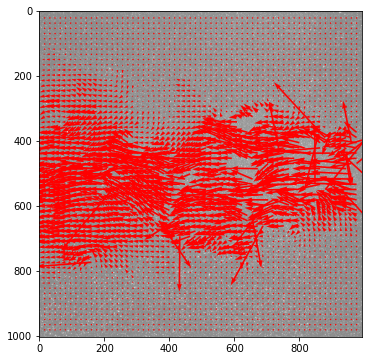## Ensemble averaged correlation using FFT based correlation from OpenPIV¶

In :
corrs = []
for i,j in zip(imlist[::2],imlist[1::2]):
# print(i,j)
corrs.append(fft_correlate_images(moving_window_array(imread(i),64,32),
moving_window_array(imread(j),64,32),
normalized_correlation=True))

In :
corrs = np.array(corrs)  # save also single image pair correlations
mean_correlation = corrs.mean(axis=0)  # ensemble average

In :
# Let's compare the result with instantaneous results
contourf(mean_correlation[23,:,:])
colorbar()

Out:
<matplotlib.colorbar.Colorbar at 0x7fcea8020970>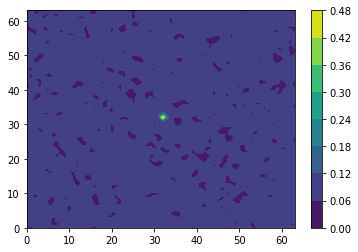In :
for i in range(corrs.shape):
figure()
contourf(corrs[i,252,:,:])
colorbar()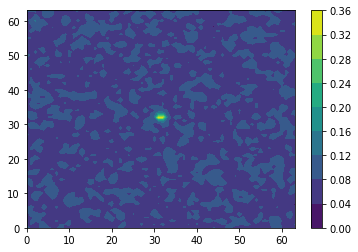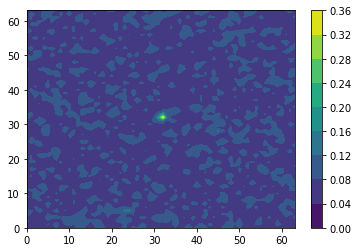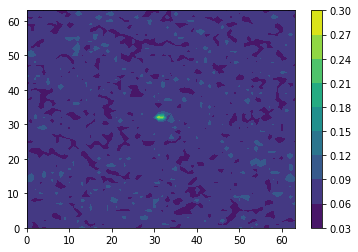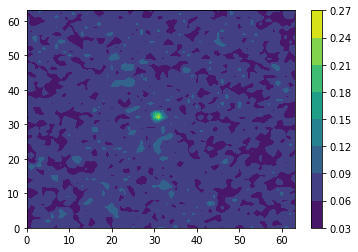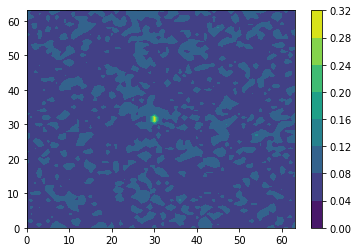In :
im = imread(imlist)
im.shape

Out:
(1004, 992)
In :
grid = get_field_shape(im.shape,search_area_size=64,overlap=32)
nrows, ncols = grid, grid

In :
u,v = correlation_to_displacement(mean_correlation, nrows, ncols)

In :
x,y = get_coordinates(im.shape, 64, 32)

In :
fig, ax = subplots(figsize=(8,8))
ax.quiver(x,y,u,v,scale=80,width=.003)
ax.invert_yaxis()
plot(u.mean(axis=1)*80+400,y[:,0])

Out:
[<matplotlib.lines.Line2D at 0x7fce9e3c5e20>]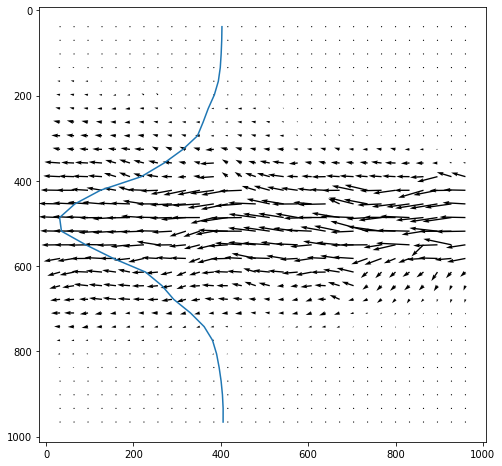In :
# another way is the averaging of velocity fields

In :
U = []
V = []

for i in range(corrs.shape):
tmpu,tmpv = correlation_to_displacement(corrs[i,:,:,:], nrows, ncols)
U.append(tmpu)
V.append(tmpv)
fig, ax = subplots(figsize=(6,6))
ax.quiver(x,y,tmpu,tmpv,scale=200)
ax.invert_yaxis()
plot(tmpu.mean(axis=1)*80+400,y[:,0])

U = np.array(U)
V = np.array(V)

meanU = np.mean(U, axis=0)
meanV = np.mean(V, axis=0)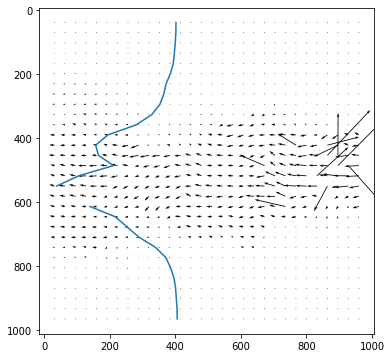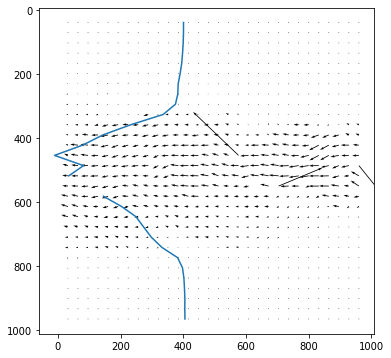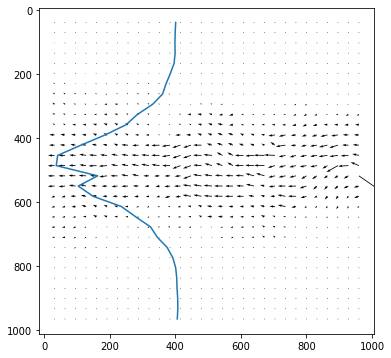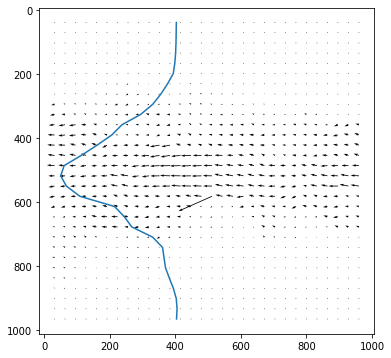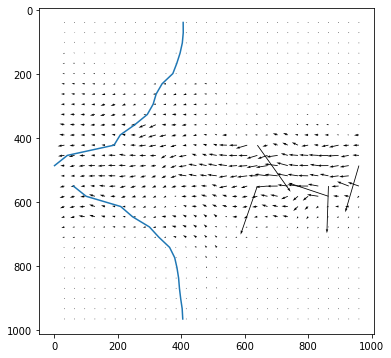In :
fig, ax = subplots(figsize=(8,8))
ax.quiver(x,y,meanU,meanV,scale=200)
ax.invert_yaxis()
plot(meanU.mean(axis=1)*80+400,y[:,0])

Out:
[<matplotlib.lines.Line2D at 0x7fcea8c6c8b0>]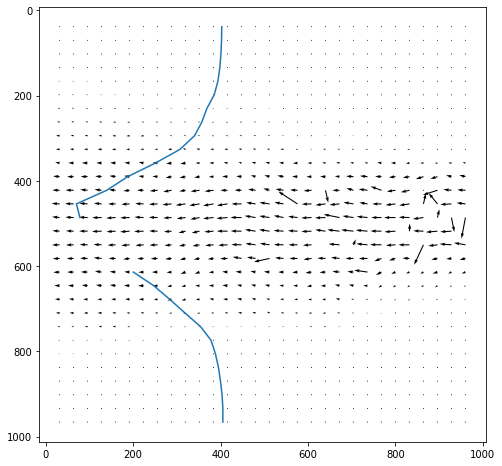In [ ]: